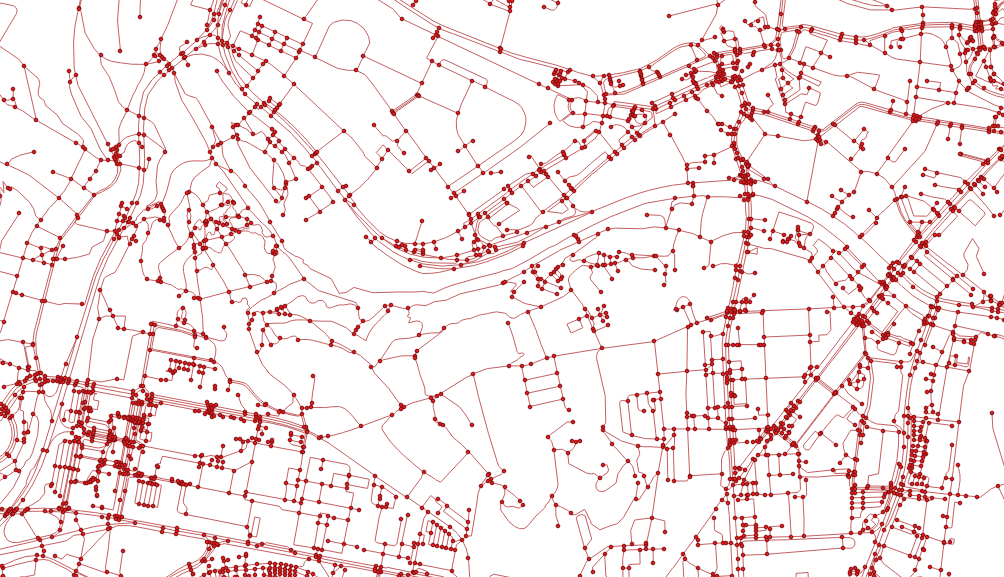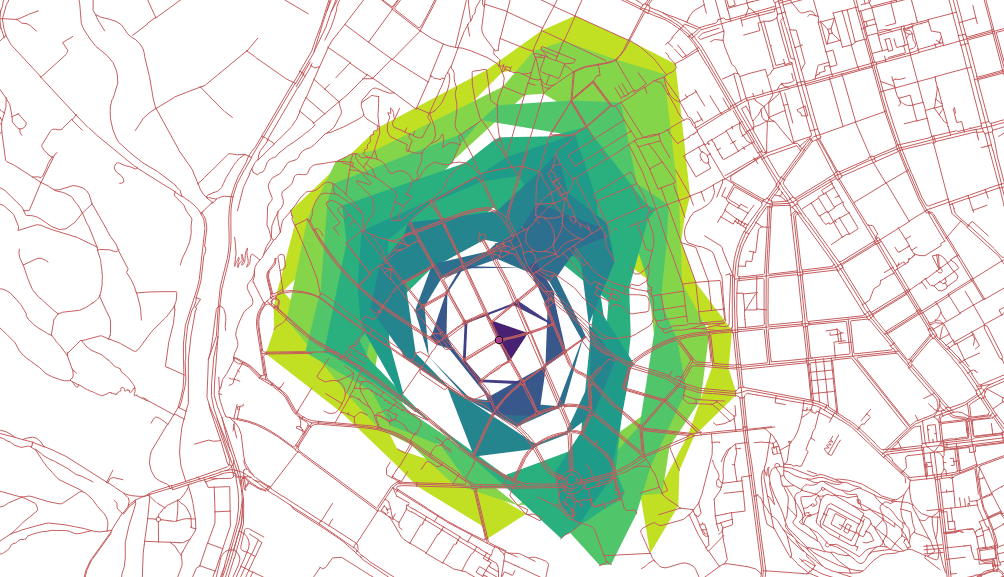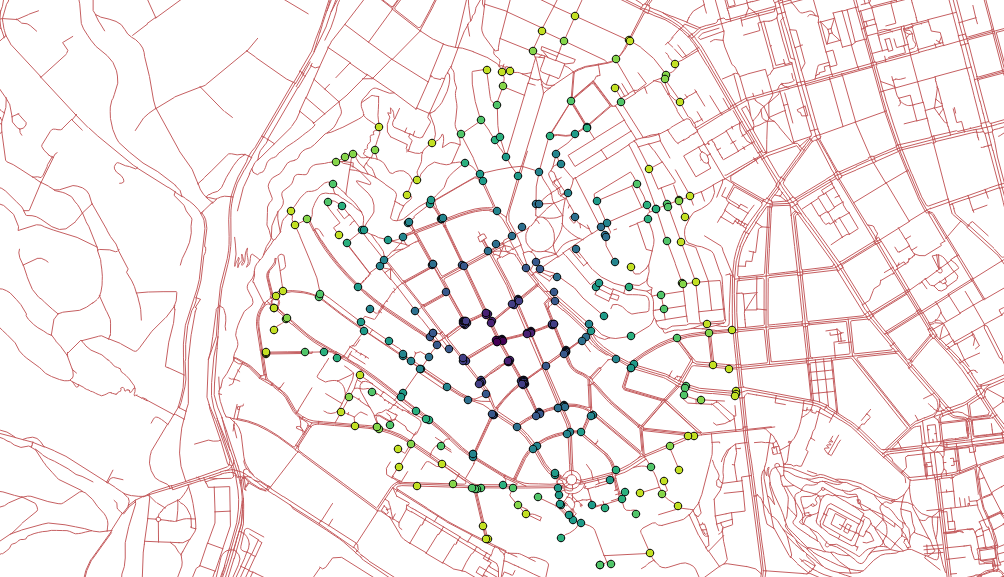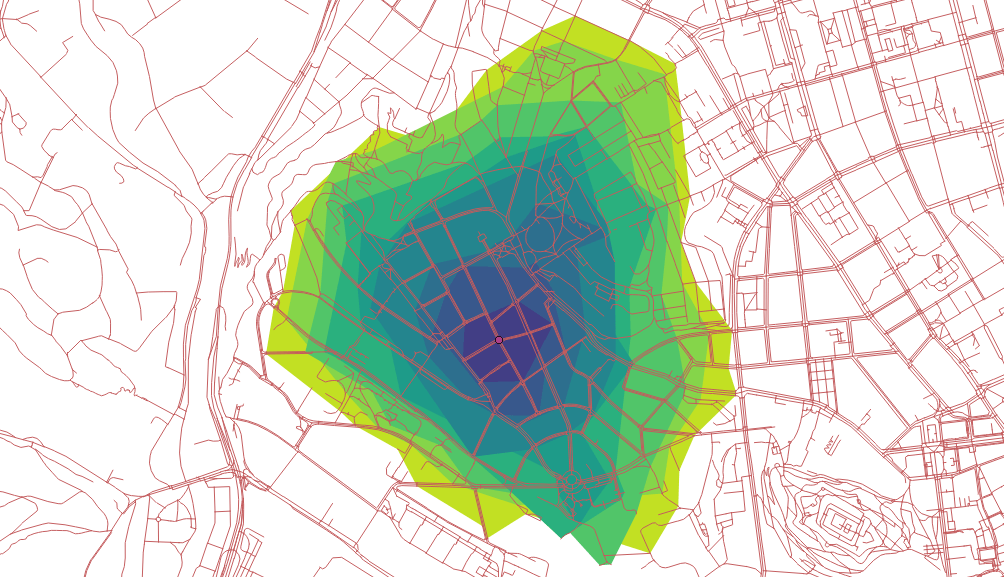# Routing with pgRouting: Catchment Area Calculation

For a long time I’ve wanted to play with pgRouting and that time has finally come. Among many other routing functions there is one that caught my eye, called `pgr_drivingdistance`. As the documentation says, it returns the driving distance from a start node using Dijkstra algorithm. The aforementioned distance doesn’t need to be defined in Euclidean space (the real distance between two points), it might be calculated in units of time, slopeness etc. How to get it going?

## Data

OSM will do as it always does. There is a tool called `osm2pgrouting` to help you load the data, the pure GDAL seems to be a better way to me though. Importing the downloaded data is trivial.

```ogr2ogr -f "PostgreSQL" PG:"dbname=pgrouting active_schema=cze" \
-s_srs EPSG:4326 \
-t_srs EPSG:5514 \
-lco GEOMETRY_NAME=the_geom \
-lco FID=id \
-gt 65000 \
-nlt PROMOTE_TO_MULTI \
-clipsrc 16.538 49.147 16.699 49.240
```

To route the network, it has to be properly noded. Although pgRouting comes with built-in `pgr_nodenetwork`, it didn’t seem to work very well. To node the network, use PostGIS `ST_Node`. Note this doesn’t consider bridges and tunnels.

```CREATE TABLE cze.roads_noded AS
SELECT
(ST_Dump(geom)).geom the_geom
FROM (
SELECT
ST_Node(geom) geom
FROM (
SELECT ST_Union(the_geom) geom
) a
) b;
```

After noding the network, all the information about speed limits and oneways is lost. If needed, it can be brought back with following:

```CREATE INDEX ON cze.roads_noded USING gist(the_geom);

SET maxspeed = a.maxspeed
FROM (
SELECT DISTINCT ON (rn.id)
rn.id,
r.maxspeed
JOIN cze.roads r ON (ST_Intersects(rn.the_geom, r.the_geom))
ORDER BY rn.id, ST_Length(ST_Intersection(rn.the_geom, r.the_geom)) DESC
) a
```

With everything set, the topology can be built.

```ALTER TABLE cze.roads_noded ADD COLUMN source integer;
```

This function creates the `cze.roads_noded_vertices_pgr` that contains all the extracted nodes from the network.As already mentioned, measures other than length can be used as a distance, I chose the time to get to a given node on foot.

```ALTER TABLE cze.roads_noded ADD COLUMN cost_minutes integer;
SET cost_minutes = (ST_Length(the_geom) / 83.0)::integer; -- it takes average person one minute to walk 83 meters

SET cost_minutes = 1
WHERE cost_minutes = 0;
```

## Routing

Now the interesting part. All the routing functions are built on what’s called inner queries that are expected to return a certain data structure with no geometry included. As I want to see the results in QGIS immediately, I had to use a simple anonymous PL/pgSQL block that writes polygonal catchment areas to a table (consider it a proof of concept, not the final solution).

```DROP TABLE IF EXISTS cze.temp;
CREATE TABLE cze.temp AS
SELECT *
JOIN (
SELECT *
FROM pgr_drivingDistance(
'SELECT id, source, target, cost_minutes as cost, cost_minutes as reverse_cost FROM cze.roads_noded',
6686,
10,
true
)
)dist ON ver.id = dist.node;

DO \$\$
DECLARE
c integer;
BEGIN
DROP TABLE IF EXISTS tmp;
CREATE TABLE tmp (
agg_cost integer,
geom geometry(MULTIPOLYGON, 5514)
);

-- order by the biggest area so the polygons are not hidden beneath the bigger ones
FOR c IN SELECT agg_cost FROM cze.temp GROUP BY agg_cost HAVING COUNT(1) &gt; 3 ORDER BY 1 DESC LOOP
RAISE INFO '%', c;
INSERT INTO tmp (agg_cost, geom)
SELECT
c,
ST_Multi(ST_SetSRID(pgr_pointsAsPolygon(
'SELECT
temp.id::integer,
ST_X(temp.the_geom)::float AS x,
ST_Y(temp.the_geom)::float AS y
FROM cze.temp
WHERE agg_cost = ' || c
), 5514));
END LOOP;
END\$\$;
```

Using `pgr_pointsAsPolygon` renders resulting nodes accessible in 10-minute walk in polygons, but weird looking ones. Not bad, could be better though.```SELECT
agg_cost,
ST_PointN(geom, i)
FROM (
SELECT
agg_cost,
ST_ExteriorRing((ST_Dump(geom)).geom) geom,
generate_series(0,ST_NumPoints(ST_ExteriorRing((ST_Dump(geom)).geom))) i
FROM tmp
) a;
```

Looks good, could be better though.How about creating concave hulls from the extracted nodes?

```SELECT
agg_cost,
ST_ConcaveHull(ST_Union(geom)) geom
FROM (
SELECT
agg_cost,
ST_PointN(geom, i) geom
FROM (
SELECT
agg_cost,
ST_ExteriorRing((ST_Dump(geom)).geom) geom,
generate_series(0,ST_NumPoints(ST_ExteriorRing((ST_Dump(geom)).geom))) i
FROM tmp
) a
) b
GROUP BY agg_cost
ORDER BY agg_cost DESC;
```

This one looks the best I guess.## Remarks

• The documentation doesn’t help much.
• I’d expect existing functions to return different data structures to be easy-to-use, actually.
• `LATERAL` might be really handy with those inner queries, have to give it a shot in the future.